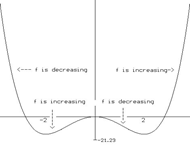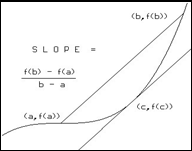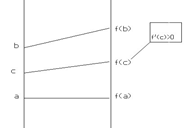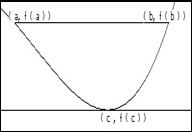CHAPTER III Applications of the Derivative (Draft- 2014 work in progress)
III.B.2 Analysis of Local ( Relative ) Extremes.
Draft Version 4/19/14

Review of extremes: We have discussed the issues of extreme values for a function $f$ previously in section I.I.3. In that section we showed that for an extreme value of a (continuous) function to occur at a point $c$ inside an interval that point would have to be a critical point for the function, i.e., either $f$ does not have a derivative at the point $c$ or $f '(c)=0$. Even if the value of a function is an extreme for an interval, it may not be the extreme value for the entire domain of the function.

A point that is an extreme for a function when considered for all points in the domain of the function is called a global (or absolute) extreme point.
A point that is an extreme for a function when considered only for points inside some interval is called a local (or relative) extreme point.

You can think about the distinction between local and global extremes in the context of a simple example like the tallest or shortest person or the highest or lowest temperature or greatest or least probability density. A local extreme may not be the global extreme for the function for its domain, but the global extremes of the function will be local extremes for the function as long as they are located inside some interval of the domain. To consider just one of the examples just mentioned, the tallest (or shortest) person in a room- a local extreme- may not be the tallest or (shortest) person in the United States or the world! - a global extreme, while the tallest (or shortest) person in the world will certainly be the tallest (or shortest) person as a member of any collection of people.

If the global extreme occurs at the endpoint of an interval in the domain, it is sometimes described as a boundary or endpoint extreme. The boundary extremes are usually distinguished from local extremes because they can be more sensitive to information that may extend a function beyond its current domain. For example, if $f(x)= x^2 + 3$ on the interval $[1,5]$, the extreme values for the function occur at the endpoints $1$ and $5$, yet if the function is extended to a longer interval $[0,6]$ the extremes would happen at $0$ and $6$. This instability would not be present for local extremes since their properties are determined by an open interval in the domain and thus their property of being a local extreme is not altered in any small change of the function's domain.

Interpretation: On the graph of a continuous function, the local extreme points are visibly prominent since they appear as the points at the top of
hills or the bottoms of valleys. In
 Something to think about: We can define two related functions giving the maximum and minimum value of a function that depend on a function and the endpoints of the interval for which the function is determined. For example we could fix the function as $f(x)= x^2$ and the interval $[-2, b]$ where $b>-2$. Then $max(b) = 4$ for $-2< b \le 2$ and $b^2$ for $b >2$ while $min(b) = b^2$ for $b\le 0$ and $0$ for $b >2$. Thus with $b > -2$ for any interval $[-2,b]$ the point $b$ will be a boundary maximum when  $2\ge b$  and a boundary minimum when $b\le 0$.  The values of local extremes will appear on the graphs of "$max$" and "$min$"  as intervals where these graph appears constant while boundary extremes will appear as sections where the graph is steadily increasing or decreasing.
other words, the function will be either increasing shortly before reaching this point and then decreasing afterwards, or vice versa. On a mapping diagram visualizing motion the local extremes are points in time where the position function changes its direction.

Determining the local extremes of a function: Since a local extreme occcurs inside an interval, the Critical Point Theorem of Chapter I.I.3 can be applied to find the local extremes of a function f. Local expremes can occur only at the critical points. So to find local extremes for f , the sensible thing to do is find the critical points of the function f. Though this is not always an easy task, the derivative calculus usually make finding the derivative, f', a mechanical task in applying the rules. The more difficult part of finding the critical points is solving the equation, f'(c)=0,that characterizes these points. After finding the critical points, the local extreme problem is reduced to determining which of the critical point is a local extreme and what kind (max or min) is it.
The following tests based on analysis of the first derivative can be used to determine the behavior of a function at a critical point inside an interval for local extremes. From the remarks about interpretations, these tests should seem sensible.

Theorem III.*** (The First Derivative Tests for Local Extrema):
If $c$ is a critical point for $f$ inside an interval $I$ and when $x < c , f '(x) < 0$ while when  $x > c, f '(x) > 0$ then $c$ is a (local or relative) minimum point for $f$.
If $c$ is a critical point for $f$ inside an interval $I$ and when $x < c , f '(x) > 0$ while when  $x > c, f '(x) < 0$ then $c$ is a (local or relative) maximum point for $f$

We visualize these situations with first derivative sign chart analysis as we did in the examples earlier in this section. See Figure 1.

 $f '(x)$  - - - - - 0 + + + + + + 0 - - - - - - - - 0 + + + +                                                                                        $x$                 $m_1$                  $M$                    $m_2$             $f$ is:     $\searrow$   f$(m_1)$    $\nearrow$       $f(M)$     $\searrow$   $f(m_2)$    $\nearrow$        Figure 1: Enhanced Sign Chart for $f'(x)$
From the derivative analysis or the test it should be clear in Figure 1 that $m_1$ and $m_2$ are local minimum points while $M$ is a local maximumpoint.

In Figure 2 the graph of a function illustrates the features of these relative extrema.

Note:  There are two cases where a critical point is not a local extreme. These are when $f '(x) >0$ for $x$ close to, but not equal to, the critical point  (in which case the function is increasing on the interval including the critical point) and when $f '(x)<0$ for $x$ close to, but not equal to, the critical point (in which case the function is decreasing on the interval including the critical point). Though these cases of critical points that are not extremes are not usually given a short description, I usually describe them as "cheep thrills", since finding a critical point can initially give the thrill of having located an extreme, but in fact that thrill is disappointed by further analysis of the derivative at nearby points.

Example: These "cheep thrill" situations are exemplified by the function f$(x) = -x^3+1$. Here $f '(x)= - 3x^2$ so $f '(x)=0$ only when $x = 0$ and otherwise $f '(x)<0$.
Thus although $x=0$ is a critical point for $f$, $f$ does not have a local extreme here. $f$ is decreasing on its domain of all real numbers. The graph of $f$ and the mapping diagram both add some meaning to the situation. It is comparable to riding a roller coaster that seems to be leveling off after a steep decline and just when you think you are going to level off fully the track proceeds downward again in an unexpected fashion. In a way, the leveling off is the critical point, and the unexpected direction is the fact that a negative derivative is consistent with the zero derivative at the critical point.

III.B.3. The Mean Value Theorem.

Theorems P and N seem so sensible from the various interpretations and examples that you may feel that they need no further justification.
One of the features of mathematics that distinguishes it amongst the sciences as a discipline is logical connections and structures that relate many statements in mathematics.
The ability to logically derive many results from a small list of statements has always made mathematical structure more convincing because of the ability to build on a foundation of a few statements that can be accepted or verified through some experience which is credible.
The foundations of calculus have been built in several different ways over the history of mathematics. One widely accepted approach developed in the eary 19th century by the French mathematician Augustin Cauchy (1789–1857) used the Mean Value Theorem as the key to justifying most of the results of the calculus known at that time.
The next two theorems are in fact equivalent in the sense that each can be proven without much difficulty assuming the other is true.
They are the most important theoretical tools used for the study of functions based on derivative analysis. After stating the results we will look at their interpretations to see why they make sense before providing a proof based on the extreme value theorem and the critical point theorem from Chapter I.I.
In the concluding part of this section we will apply the Mean Value Theorem to justify more rigorously (prove) the results of first derivative analysis of increasing and decreasing functions.

 Theorem III.B.3. The Mean Value Theorem: (MVT) If $f$ is continuous on $[a,b]$ and differentiable on $(a,b)$ then there is some number $c$ where $a < c < b$ and $f '(c) = \frac{f(b) - f(a)}{b-a}$                     or  $f(b) = f(a) + f '(c)\cdot (b-a)$.

Interpretations: 1. Motion. Suppose $f(t)$ is the position of an object moving continuously over a time interval $[a,b]$ with a velocity at every moment between time$a$ and $b$.  The MVT can be interpreted in this situation as meaning that at some moment in time $c$ between time $a$ and $b$ the instantaneous velocity of the object will be equal to the average velocity of the object over the interval $[a,b]$.
2. Graphical. Consider the graph of the function $f$ over the interval $[a,b]$. The MVT can be interpreted in this situation as meaning that for some $c$ between $a$ and $b$, the slope of the tangent line at that point will be equal to the slope of the (secant) line between the end points of the graph $(a,f(a))$ and $(b,f(b))$. To express it geometrically, at some point on the graph of $f$ the tangent line will be parallel to the secant line determined by the endpoints. See Figure III.B.3.
3. Economics. Suppose $P$ is a differentiable profit function for producing a commodity measured by the variable $x$ at levels of production between $a$ and $b$. If the average profit per additional unit of production for this range of production is $P*$ then the MVT can be interpreted to mean that at some level of production between $a$ and $b$ the marginal profit will be $P*$.
4. Probability. Suppose $F$ is the probability distribution for a continuous random variable $X$. When $F$ is differentiable for $X$ between $A$ and $B$ the MVT means that the average probability density that $X$ is between $A$ and $B$ is equal to the point probability density of $X$ at some point $C$ between $A$ and $B$.
5. Estimates. In considering estimates of $f(b)$ based on $f(a)$, there is a point $c$ between $a$ and $b$ where the differential at $c$ using $dx=b-a$, $df(c,b-a) = f'(c) (b-a)$, will be precisely the difference, $f(b)-f(a)$. Thus for example, if the values of the derivative of $f$ between $a$ and $b$ are always greater than $f'(a)$, then the differential at $a$ will be less than the differential at $c$, and thus underestimate the difference $f(b)-f(a)$. In other words, $f(b)-f(a) > df(a,b-a)$ and so $f(b) > f(a) + df(a, b-a)$.

III.B.4. Application of the MVT to first derivative analysis.

Let's defer the proof of the Mean Value Theorem till later and see how we can use it to justify Theorem P, i.e., if the derivative of a function is positive on an interval then the function is increasing on that interval.
 Theorem III.B.1. (P) If $f$ is a differentiable function on an interval $I$ with $f '(x) > 0$ for all $x$ in the interval, then $f$ is increasing on the interval. If $f '(x) \ge 0$ for all $x$ in the interval, then $f$ is non-decreasing on the interval. Theorem III.B.2. (N) If $f$ is a differentiable function on an interval $I$ with $f '(x) < 0$ for all $x$ in the interval, then $f$ is decreasing on the interval. If $f '(x)\le 0$ for all $x$ in the interval, then $f$ is non-increasing on the interval.

[We'll leave the proof of theorem N for the reader to supply by following the argument for Theorem N.]
Proof of Theorem P: Recall the hypothesis of Theorem P is that f$'(x) > 0$ for all $x$ in the interval $I$. To show that $f$ is increasing on the interval $I$ we consider two distinct points, $a$ and $b$, in the interval. For convenience we will assume that $a < b$.
We want to show is that for these arbitrarily chosen points, $f(a)<f(b)$. See Figure ***.
Since $f$ is assumed differentiable on the interval $I$, $f$ is continuous on $[a,b]$ and differentiable on $(a,b)$.We apply the MVT to $f$ on $[a,b]$ to conclude there is some number $c$ in $(a,b)$ {and hence in $I$ } where
$f '(c) = \frac{f(b) - f(a)}{b-a}$
In other words $f(b) = f(a) + f'(c) \cdot (b-a)$.
From the assumptions , $f'(c) > 0$ and  $b - a > 0$ , so in the last expression it must be that $f'(c)\cdot (b- a)>0$ , so  $f(b) > f(a)$.
In summary, if $a < b$ in $I$  then $f(a) < f(b)$, so $f$ is an increasing function on $I$.             EOP

Interpretations of the argument in the proof of Theorem P:
1. Motion. If the instantaneous velocity is always positive, then the average velocity must be positive (MVT)  for any interval, which means the position is increasing when compared at any two points in time.
2. Graphical. If the slopes of the tangent lines are always positive, then the slope of any secant line must be positive (MVT) and hence the graph of the function is always rising between any two points on the graph.
3. Economic. If marginal profit is positive at any level of production in the interval, then for any increase in production the average profit per unit increase will also be positive and so the profit will also increase.

It is now time to prove the Mean Value Theorem.

Proof of the Mean Value Theorem:  The idea of this proof can be thought of graphically as comparing the function $f$ with the linear function that passes through $(a,f(a))$ and $(b,f(b))$ and has slope $\frac{f(b) - f(a)}{b-a}$. For the remainder of the argument we will denote the slope by $M$. We consider the difference of these two functions and notice from the figure which we use to visualize the situation that this difference seems to be greatest precisely at the point where the tangent line is parallel to the secant line.
To discuss the difference we let $g(t) = f(a) + M \cdot (t - a) - f(t)$ for all $t$ in $[a,b]$.

Since $f$ and the linear function are continuous on $[a,b]$ and differentiable on $(a,b)$, $g$ is continuous on $[a,b]$ and differentiable on $(a,b)$ as well. By the continuity of $g$ we can apply the extreme value theorem to say that for some $c_*$ and $c^*$ in $[a,b]$ we have extreme values for $g$ on the interval. If either of these points is inside the interval then we can apply the critical point theorem to $g$ to say that $g'(c_*) = 0$ or $g'(c^*)= 0$.

But when we compute we find $g'(t) = M - f'(t)$.  Thus we have either $M - f'(c_*) = 0$, in which case $f'(c_*)= 0$, or $M - f'(c^*) = 0$,  in which case $f'(c^*) = M$. This means that if either $c_*$ or $c^*$ are in the open interval $(a,b)$ the theorem's conclusion is satisfied.
This leaves only the case when the endpoints, $a$ and $b$ are the points where the extremes of $g$ occur.  Now we evaluate $g$ at these two points.
$g(a) = f(a) + M\cdot (a - a) - f(a) = 0$    while $g(b) = f(a) + M\cdot(b - a) - f(b) = f(a) + \frac {f(b)-f(a)}{b-a} \cdot (b - a) - f(a) = 0$.
But if both the maximum and minimum values of $g$ are 0, then $g(t) = 0$ for all $t$ in $[a,b]$.
Looking back at the definition of $g$, we see that in this case the last equation means that $f(t)=f(a) + M \cdot (t - a)$ for all $t$ in $[a,b]$.
Thus we conclude that in this case $f'(c) = M$ for not just some, but for any $c$ in $[a,b]$.

In summary , we see that in any case the conclusion of the Mean Value Theorem is satisfied at some point $c$ in $(a,b)$.      EOP

 Here is a GeoGebra applet that visualizes some aspects of the proof . It also provides with a dynamic tool that allows you to investigate lines parallel to the secant line to see how these lines can be moved up or down to find the number $c$ that exists according to the theorem where $f '(c) = \frac{f(b) - f(a)}{b-a}$.

The next theorem is sometimes proven before the Mean Value Theorem even though as you can see it is merely a special case of the MVT.Theorem III.B.4. Rolle's Theorem: If $f$ is continuous on $[a,b]$ and differentiable on $(a,b)$ and $f(b) = f(a)$ then there is some $c$ where $a < c < b$ and $f'(c) = 0$  .

Proof of Rolle's Theorem assuming the MVT:  In Rolle's theorem the assumptions of the MVT are all assumed along with the added assumption that $f(b) = f(a)$. This last assumption implies that $f(b) - f(a) = 0$ , so by applying the MVT to $f$ gives the claimed result.

Interpretations: 1. Motion. Suppose $f(t)$ is the position of an object moving continuously over a time interval $[a,b]$ with a velocity at every moment between time $a$ and $b$. The assumptions for Rolle's Theorem also assume that $f(a) = f(b)$, i.e., from the point of view of motion at times $a$ and $b$, there seems to be no change in position. Rolle's Theorem can be interpreted in this situation as meaning that at some moment in time $c$ between time $a$ and $b$ the instantaneous velocity of the object will be equal to $0$, i.e. , at the instant $c$ the object appeared to be stopped.
2. Graphical. Consider the graph of the function $f$ over the interval $[a,b]$. Here the assumption that $f(a) = f(b)$ means that the secant line between $(a,f(a))$ and $(b,f(b))$ is horizontal with its slope $0$. Rolle's Theorem can be interpreted in this situation as meaning that for some $c$ between $a$ and $b$, the slope of the tangent line at that point will be $0$. To express it geometrically, the tangent line will be horizontal at some point on the graph of $f$ . See Figure ***.
3. Economic. If the profit at two levels of production, $a$ and $b$,are equal, then at some level of production between those $a$ and $b$, the marginal profit is $0$.

Exercises III.B.
In exercises 1-6, for each of the functions find  the intervals where the function is increasing and decreasing.
1.    $f(x) = x^2 - 4x + 3$
2.    $f(x) = 2 x^3 + 3 x^2 + 4$
3.    $g(t) = t^3 - 3 t + 4$
4.    $g(t) = \sin(t) + \sqrt 3 \cos(t)$
5.    $r(s) = s + \frac 1s$
6.    $p(x) = x^4 - 2 x^2 + 2$
For exercises 7-12, for each of the functions in exercises 1 - 6, find the local extreme points and values. Use this information together with the original information found in those problems to sketch the graphs of these functions. If possible, compare your results with the graph from some graphing calculator or computer graphing system.
13. Use the first derivative to graph  the following functions, giving the coordinates of any (local) extremes:
a. $f(x) = x + 2 \sin(x)$       b. $f(x) = \frac x{x^2 + 1}$
14. Suppose $f(x)$ is differentiable  and $f'(x) = 0$ only when $x = 2$ and $4$. Describe all possible shapes for the graph of $f$. Explain your reasoning and give an example of such a function.
15. Explain why a cubic polynomial can have at most two local extrema.
16. Explain why a quartic polynomial can have at most three local extrema.
17. Generalize your results in exercises 15 and 16 to polynomials of degree $n$. Explain.
18. Find all the local extreme points for the sine function. Explain why the sine function cannot be a polynomial function.
19. Project. A function $f$ is called an even function if $f(-x) = f(x)$ for all $x$ in its domain. Discuss the implications of $f$ being an even function for the mapping diagram and the graph of $f$. Suppose $0 < a < b$ .
Prove that if $f$ is differentiable and  an even function then $f$ is increasing on the interval $(a,b)$ if and only if $f$ is decreasing on the interval $(-b,-a)$.
20. Project. A function $f$ is called an odd function if $f(-x) = -f(x)$ for all $x$ in its domain. Discuss the implications of $f$ being an odd function for the mapping diagram of $f$ and the graph of $f$. Suppose $0 < a < b$.
Prove that if $f$ is differentiable and  an odd function then $f$ is increasing on the interval $(a,b)$ if and only if $f$ is increasing on the interval $(-b,-a)$.